# Frank Solutions for Chapter 11F Carboxylic Acid Class 10 Chemistry ICSE

1. Write the molecular formulae and structure formula of Ethanoic acid ?

2. What is vinegar and glacial acetic acid ?

Vinegar : Vinegar is 4 to 6 % acetic acid (Ethanoic Acid).
Glacial acetic acid : Pure acetic acid is called glacial acetic acid.

3. Write three physical properties of acetic acid.

Three physical properties of acetic acid :

• State: Liquid
• Odour: Pleasant smell - smell of vinegar
• Taste: Sour taste

4. Write the important uses of acetic acid.

Uses of acetic acid:

• As a solvent for gums, resin, cellulose etc.
• As a laboratory reagent
• In medicines
• As a vinegar for table purpose and for manufacturing pickels
• For making rubber, rayon, plastic, varnishes etc.
• For the manufacture of dyes, perfumes, pigments.

5. What is the main constituent of vinegar ?

Acetic acid is the main constituent of vinegar .

6. What is the result of oxidation of ethyl alcohol?

oxidation of ethyl alcohol gives acetic acid.

7. What is the action of acetic acid with litmus paper ?

Acetic acid turns blue litmus red. It proves that it is acidic in nature.

8. What is the boiling point of acetic acid?

Boiling Point : 118°C

9. Define glacial acetic acid?

Pure acetic acid is called Glacial acetic acid because it forms an ice- like solid when cooled.

10. Name the first four members of aliphatic carboxylic acid.

The first four members of aliphatic carboxylic acid are :

• Methanoic acid
• Ethanoic acid
• Propanoic acid
• Butanoic acid

11. How will you carry out the following conversions ?
(i) Ethene to acetic acid
(ii) Ethane to acetic acid

12. Fill in the blank with appropriate word/words.
(i) Vinegar is a solution of about _____ per cent ______in water.
(ii) The general formula for alkene and alkyne are _____ and____respectively
(iii) The catalyst used for hydrogen of vegetable oil is ______
(iv) For artificial ripening of fruits _____is used
(v) Bayer's reagent is ______
(vi) Esterification is the reaction between carboxylic acid and ____ in presence of ____
(vii) Denatured alcohol is a mixture of _____ and ____
(viii) For test of unsaturation _____is used
(ix) In laboratory ethyne is prepared by the reaction of water an _____
(x) Two isomers of the compound having molecular formula C3H6O are _____and _____

(i) Vinegar is a solution of about 4 to 6 per cent acetic acid in water.
(ii) The general formula for alkene and alkyne are CnH2n and CnH2n-2,respectively.
(iii) The catalyst used for hydrogen of vegetable oil is nickel.
(iv) For artificial ripening of fruits, ethylene is used.
(v) Bayer's reagent is alkaline KMnO4.
(vi) Esterification is the reaction between carboxylic acid and alcohol in the presence of conc. H2SO4.
(vii) Denatured alcohol is a mixture of ethyl alcohol and methanol.
(viii) For test of unsaturation, bromine water is used.
(ix) In the laboratory, ethyne is prepared by the reaction of water and calcium carbide.
(x) Two isomers of the compound having molecular formula C3H6O are propanal and propanol.

13. (i) Compounds having the same molecular formula but different properties are called
(a) Isotopes
(b) Isomers
(c) Isobars
(d) Allotrops
(b) Isomers

(ii) The first organic compound prepared by Wohler in the laboratory was
(a) acetic acid
(b) methane
(c) Acetylene
(d) Urea
(d) Urea

(iii) Soda-lime is a mixture of
(a) NaOH + CaCl2
(b) NaOH + CaCO3
(c) NaOH + CaO
(d) NaOH + Al2O3
(c) NaOH + CaO

(iv) When sodium propionate is heated with soda-lime, the product formed is
(a) Methane
(b) Ethane
(c) Ethene
(d) Ethyne
(b) Ethane

(v) LPG contains
(a) Methane
(b) Ethane
(c) Butane
(d) None of these
(c) Butane

(vi) Methane reacts with energy of chlorine in diffused sunlight to give the final product as
(a) Chloroform
(b) Carbon tetrachloride
(c) Methylene chloride
(d) Methyl chloride
(b) Carbon tetrachloride

(vii) Ethene reacts with 1% cold alkaline KMnOto give
(a) Oxalic acid
(b) Ethyl alcohol
(c) Acetaldehyde
(d) Ethylene glycol
(d) Ethylene glycol

(viii) When ethanol is heated with conc. H2SO4 at 170oC, it gets converted into ethane. In this reaction conc. H2SO4 acts as
(a) Oxidizing agent
(b) Catalyst
(c) Dehydrating agent
(d) Reducing agent
(c) Dehydrating agent

14. (i) For each of the compounds : (a) Ethane (b) Vinegar and (c) Marsh gas draw the relevant structural formula.
(ii) What word is used to describe these three compounds taken together ?

(i) Structural formula are as under :
(a)  Ethane(ii) The three compounds taken together are known as Organic compounds.

15. (i) What is the special feature of the structure of :
(a) C2H2 (b) C2H4
(ii) What type of reaction is common to both of these compounds ?

(i) (a) The special feature of the structure of C2H2 is that there is presence of triple bond in the molecule.
(b) The special feature of the structure of C2H2 is that there is presence of double bond.
(ii) Addition reaction is common to both of these compounds.

16. Give the name and structural formula of :
(i) A Saturated Hydrocarbon
(ii) An unsaturated hydrocarbon with a double bond.

(i) The name of saturated Hydrocarbon is called Alkane and the formula is CnH2n+2 where n = 1,2,3.......
(ii) The name of unsaturated hydrocarbon with double bond is called alkene and the formula is CnH2n , where n = 1,2,3.......

17. Copy and complete the following sentence :
A saturated hydrocarbon will undergo  ______ reactions, whereas the typical reaction of an unsaturated hydrocarbon is ______

A saturated hydrocarbon will undergo Substitution reactions, whereas the typical reaction of an unsaturated hydrocarbon is Addition

18. (i) Write the equation for the laboratory preparation of Ethyne (acetylene) from calcium carbide.
(ii) What is the special feature of the structure of Ethyne?
(iii) What would you see when Ethyne is bubbled through a solution of bromine In carbon tetrachloride?
(iv) Name the addition product formed between ethane and water.(ii) Special feature of the structure of Ethyne is that there is presence of triple bond.
(iii) When Ethyne is bubbled through a solution of bromine in carbon tetrachloride, the orange colour of bromine disappears due to formation of colourless product.
(iv) Ethyl alcohol is formed when the addition reaction takes place between ethene and water.19. State what do you observe when ethene is bubbled through a solution of bromine in tetrachloromethane (carbon tetrachloride).

When ethene is bubbled through a solution of bromine in tetrachloromethane, the orange colour of bromine disappears due to the formation of colourless ethylene bromide.20. Fill in the blanks by choosing words from the given list of words :
Addition, Carbohydrates, CnH2n-2 , CnH2n+2  ,  electrochemical, homologus, hydrocarbons, saturated, substitution , unsaturated
The  alkanes form a _____ series with general formula _____ The alkanes are _____, which generally undergo _____ reactions.

The alkanes form a homologus series with general formula CnH2n-2 . The  alkanes are saturated, which generally undergo substitution  reactions.

21. Ethanol can be converted to ethene which can then be changed to ethane.
Choose the correct word or phrase from the brackets to complete the following sentences:
(i) The conversion of ethanol to ethene is an example of  ________  (dehydration, dehydrogenation)
(ii) Converting ethanol to ethene requires the use of _______  (Concentrated hydrochloric acid, concentrated nitric acid, concentrated sulphuric acid).
(iii) The conversion of ethene to ethane is an example of ______( hydration, hydrogenation).
(iv) The catalyst used In the conversion of ethene to ethane is commonly ______ (iron, cobalt, nickel).

(i) The conversion of ethanol to ethene is an example of dehydration.
(ii) Converting ethanol to ethene requires the use of concentrated sulphuric acid.
(iii) The conversion of ethene to ethane is an example of hydrogenation.
(iv) The catalyst used in the conversion of ethene to ethane is commonly nickel

22. Write down the equation for the preparation of ethyne from calcium carbide?

23. (i) What type of reaction takes place between ethane and chlorine to form monochloro ethane ?
(ii) The reaction between ethene and chlorine forms only one product. Name the type of the reaction.
(iii) (a) Draw the structural formula of ethene.
(b) What is the feature of the ethene structure, which allows ethene to react with chlorine in the way it does ?

(i) Substitution reaction takes between ethane and chlorine to form monochloroethane. This reaction is called chlorination.
(ii) Addition reaction takes place between ethene and chlorine and it is called halogenations.
(iii) (a) Structural formula of Ethene :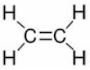(b) Ethene can react with chlorine because there is presence of double bond which can result in the addition reaction.

24. The list of some organic compounds is given below:
Ethanol, ethane, methane, ethyne and ethane
From the above list, name a compound:
(i) Formed by the dehydration of ethanol by concentrated sulphuric acid.
(ii) Which forms methanoic acid on oxidation in the presence of copper at 200°C.
(iii) Which has vapour density 14 and turns acidic potassium permanganate colourless ?
(iv) Which forms chloroform on halogenations in the presence of sunlight ?
(v) Which decolourises bromine solution in carbon tetrachloride ?

(i) Ethene
(ii) Methane.
(iii) Ethene
(iv) Methane
(v) Ethyne, Ethene

25. Write balanced equations to show the preparation of the following :
(i) Ethane from sodium propionate
(ii) Ethene from ethanol
(iii) Ethyne from Calcium carbide
(iv) Ethanoic acid from ethane

26. (i) Which compound should be heated with soda lime to obtain ethane gas in the laboratory? (ii) Write the equation for the reaction involved in the above question.
(iii) Write the balanced equation for the complete combustion of ethane.
(iv) Name a solid which can be used, Instead of conc. H2SO4 to prepare ethylene by dehydration of ethanol.
(v) Name a reagent which can be used to distinguish between ethane and ethene.
(vi) Ethylene forms  an addition product with chlorine. Name this addition product and write its structural formula.

(i) Sodium propionate is heated with soda lime to obtain ethane gas in the laboratory.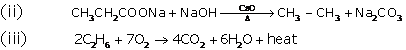(iv) Al2O3 can be used instead of sulphuric acid to prepare ethylene by dehydration of alcohol.
(v) Bromine solution can be used to distinguish between ethane and ethane.
(vi) Ethylene reacts with chlorine to form a product called as 1, 2 - dichloroethane. This reaction is known as Halogenation.27. Write balanced equation for the following :
Ethane is burnt in air.
(a) Write the equation for the preparation of ethylene from ethyl alcohol.
(b) Write the general formula for a saturated hydrocarbon and give one example of a saturated hydrocarbon with its structural formula.
(c) Name a compound which will give acetylene gas, when treated with water.

28. (a) Draw the structural formula of a compound with two carbon atoms in each of the following cases:
(i) An alkane with a carbon to carbon single bond.
(ii) An alcohol containing two carbon atoms
(iii) An unsaturated hydrocarbon with carbon to carbon triple bond.

(b) Ethane, Ethene, Ethanoic acid, Ethyne, Ethanol
From the compounds given above, name:
(i) The compound with -OH as apart of its structure.
(ii) The compound with -COOH as apart of its structure.
(iii) Homologue of homologous series with general formula CnH2n-2 .

29. Write the equations for the following laboratory preparations :
(i) Ethane from sodium propionate
(ii) Ethene from iodoethane
(iii) Ethyne from calcium carbide.

(i) Ethane from sodium propionate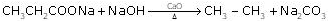(ii) Ethene from iodoethane
CH3 – CH2I + KOH → CH2 = CH2 + KI + H2O
(iii) Ethyne from calcium Carbide
CaC2 + 2H2O → C2H2 + Ca(OH)2

30. Define the term catenation.

Carbon has the unique property of combining any number of carbon atoms to form straight chains, branched chains and rings of different sizes. This property is called catenation.

31. Give the correct IUPAC name and the functional group for each of the compounds whose structural formulae are given below :
(i)

(i) IUPAC Name : Propanal
Functional group : Aldehyde
(ii) IUPAC Name : Propan-1-ol
Functional group : Alcohol

32. (i) Write the equation for the preparation of carbon tetrachloride from methane.
(ii) Draw the structural formula of ethyne.
(iii) How is the structure of alkynes different from that of alkenes ?

33. Fill in blanks with correct words from the brackets :
Alkenes are the  (i) ______ (analogous/homologous) series of (ii)  _______ (saturated/unsaturated) hydrocarbons. They differ from alkanes due to the presence of (iii) ______ (double/single) bonds. Alkenes mainly undergo (iv) ______ (addition/substitution) reactions.

(i) Homologous
(ii) Unsaturated
(iii) Double

34. Give the IUPAC names of the following compounds numbered (i) to (v). The IUPAC names of the compounds on the left are to guide you into giving the correct names of the compounds on the right.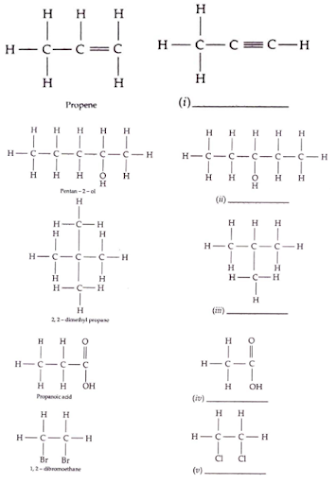(i) Propyne
(ii) Pentan - 3 - ol
(iii) 2 - methylpropane
(iv) Ethanoic acid
(v) 1, 2 - dichloroethane

35. Copy and complete the following table which relates to three homologus series of hydrocarbons :

 General formula CnH2n CnH2n+2 CnH2n+2 IUPAC name of the homologus series Characteristic bond type Single bond IUPAC name of the first member of the series Type of reaction with chlorine Addition

 General formula CnH2n CnH2n+2 CnH2n+2 IUPAC name of the homologus series Alkenes Alkynes Alkanes Characteristic bond type Double bond Triple bond Single bond IUPAC name of the first member of the series Ethene Ethyne Methane Type of reaction with chlorine Addition Addition Substitution

36. Choose the correct answer :

The formation of 1, 2- dibromoethane from ethene and bromine is an example of  :
(a) Substitution
(b) Dehydration
(c) Dehydrohalogenation

37. Name the organic compound prepared by each of the following reactions :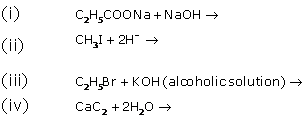38. Write the equation for the following reaction :
(i) Bromoethane and an aqueous solution of hydroxide
(ii) Calcium carbide and water
(iii) Ethene and water (steam)

39. (a) Distinguish between the saturated hydrocarbon ethane and the unsaturated hydrocarbon ethene by drawing their structural formulae.
(b) Addition reactions and substitution reactions are types of organic reactions. Which type of reaction is shown by?
(i) Ethane         (ii) Ethene?
(c ) (i) White the equation for the complete combustion of ethane.
(ii) Using appropriate catalysts, ethane can be oxidized to an alcohol, an aldehyde and an acid. Name the alcohol, aldehyde and acid formed when ethane is oxidized.
(d) (i) Why is pure acetic acid also known as glacial acetic acid ?
(ii) What type of compound is formed by the reaction between acetic acid and an alcohol?

40. Choose the correct answer :

(i) Which of the following statements is wrong about alkanes?
(a) They are all saturated hydrocarbon.
(b) They can undergo addition as well as substitution reaction.
(c) They are almost non-polar in nature.
(d) On complete combustion give out carbon dioxide and water.
(b) Statement is wrong. They can undergo addition as well as substitution reaction.

(ii) Select the acid which contains four hydrogen atoms in it.
(a) Formic acid
(b) Sulphuric Acid
(c) Nitric acid
(d) Acetic acid
(d) Acetic acid contains four hydrogen atoms in it.

41. Write a fully balanced equation for the following case :
Acetic acid is warmed with ethanol in the presence of concentrated sulphuric acid.

42. Methane is the first member of alkane, when it is treated with excess of chlorine in the presence of diffused forms carbon tetrachloride. Draw the appropriate structural formula of carbon tetrachloride and state the type of bond present is it.

Structural Formula of carbon tetrachloride :The bond is Covalent Bond.

43. Give chemical equation for :
(a) The laboratory preparation of methane from sodium acetate.
(b) The reaction of one mole of ethene with one mole of chlorine gas.
(c) The preparation of ethyne from 1, 2 - dibromoethane.

44. State how the following conversions can be carried out :

(a) Ethyl chloride to Ethyl alcohol
(b) Ethyl chloride to Ethene
(c) Ethene to Ethyl alcohol
(d) Ethyl alcohol to Ethane

45. (a) Define isomerism.
(b) Give the IUPAC name of the isomer C4H10 which has a branched chain.

(a) Compounds having the same molecular formula, but different structural formula are called isomers and the phenomenon is called isomerism.
(b) IUPAC name of branched chain isomer of C4H10 is 2 - methyl propane.

46. Select the correct answer from the choices a, b, c and d which are given.

(i) An organic compound undergoes addition reactions and gives a red colour precipitate with ammonial cuprous chloride. Therefore, the organic compound could be:
(a) Ethane
(b) Ethene
(c) Ethyne
(d) Ethanol
(c) Ethyne

(ii) The organic compound mixed with ethanol to make it spurious is :
(a) Methanol
(b) Methanoic acid
(c) Methanal
(d) Ethanoic acid
(a) Methanol

47. Draw the structural formula for each of the following:
(a) Ethanoic acid
(b) But-2-yne

48.  Compound A is bubbled through bromine dissolved in carbon tetrachloride and the product is CH2Br - CH2Br.(i)  Draw the structural formula of A.
(ii)  What type of reaction has A undergone?
(iv) Name (not formula) the compound formed when steam reacts with A in the presence of phosphoric acid.
(v) What the procedure for converting the product of (b) (IV) back to A?

(i) H2C = CH2
(iii) Bromine solution gets decolourised.
(iv) Ethanol
(v) By heating it (ethanol) with concentrated sulphuric acid at 170oC.

49. Give reason as to why :
(i) Almost 90% of all known compounds are organic in nature
(ii) It is dangerous to burn methane in an insufficient supply of air.

(i) Carbon can form a large number of compounds because of tetravalency and catenation.
(ii) In insufficient supply of air, methane burns to produce carbon monoxide which is a toxic gas.

50. Choose the correct answer from the options given below :
(i) The number of C-H bonds in ethane molecule are :
(a) Four
(b) Six
(c) Eight
(d) Ten
(b) Six

(ii) The functional group present in acetic acid is :
(a) Ketonic (C = 0)
(b) Hydroxyl (- OH)
(c) Aldehydic (- CHO)
(d) Carboxyl (-COOH)
(d) Carboxyl (-COOH)

(iii) The unsaturated hydrocarbons undergo:
(a) A substitution reaction
(b) An oxidation reaction
(d) None of the above

51. Choose the correct word/phrase from within the brackets to complete the following sentences :
(i) The catalyst used for conversion of ethane to ethane is commonly ________ {nickel/iron/cobalt)
(ii) When acetaldehyde us oxidized with acidified potassium dichromate, it forms _________ (ester/ethanol/acetic acid)
(iii) Ethanoic acid reacts with ethanol in presence of concentrated H2SO4 so as to form a compound and water. The chemical reaction which takes place is called ______ (dehydration/hydrogenation/esterification)
(iv) Write the equation for the reaction taking place between 1, 2-dibromoethane and alcoholic potassium hydroxide.
(v) The product formed when ethane gas reacts with water in the presence of sulphuric acid is __________ (ethanal/ethanol/ethanoic acid)

(i) Nickel
(ii) Acetic acid
(iii) Esterification

52. Write balanced chemical equation for the following :

(i) Monochloroethane is hydrolysed with aqueous KOH
(ii) A mixture of sodalime and sodium acetate is heated
(iii) Ethanol under high pressure and low temperature is treated with acidified potassium dichoromate
(iv) Water is added to calcium carbide
(v) Ethanol reacts with sodium at room temperature

53. Give the structural formulae for the following :
(i) An isomer of n-butane
(ii) 2-propanol
(iii) Diethyl ether

(i) An isomer of n-butane
(ii) 2-propanol
(iii)Diethyl ether

54. Give reasons for the following :
(i) Methane does not undergo addition reaction, but ethane does
(ii) Ethyne is more reactive than ethane
(iii) Hydrocarbons are excellent fuels

Reasons:
(i) Alkanes are saturated, i.e. they do not have a carbon-to-carbon double bond, and so do not undergo an addition reaction. Alkenes are said to be unsaturated because of the carbon-to-carbon double bond in their structure, and the double bond in alkenes makes them more reactive than alkanes.
(ii) Ethyne is a highly reactive compound due to the presence of a triple bond between its two carbon atoms.
(iii) Hydrocarbons are used as fuels because they burn in air producing a lot of heat energy.

55. Give balanced equation for the laboratory preparations of the following organic compounds:
(i) A saturated hydrocarbon from iodomethene
(ii) An unsaturated hydrocarbon from an alcohol
(iii) An unsaturated hydrocarbon from calcium carbide
(iv) An alcohol from ethyl bromide

Equations:
(i) CH3I + 2[H] → CH4 + HI
Iodo Methane
(ii) CH3CH2OH + H2SO4→ CH3CH2HSO4 + H2O
CH3CH2HSO4→ CH2=CH2 + H2SO4
(iii) CaC2 + 2H2O → Ca(OH)2 + C2H2
(iv) C2H5Br + NaOH → C2H5OH + NaBr

56. Identify this statement that is incorrect about alkanes.
(a) They are hydrocarbons
(b) There is a single covalent bond between carbon and hydrogen
(c) They can undergo both substitution as well as addition reactions
(d) On complete combustion they produce carbon dioxide and water

(c) They can undergo both substitution as well as addition reactions.

57. (a) Identify the gas evolved when sodium propionate is heated with sodalime
(b) Give a chemical term for a reaction in which hydrogen of a alkane is replaced by a halogen

(a) Ethane
(b) Substitution

58. Give a chemical test to distinguish between ethane gas and ethane gas.

Ethene gas decolourises the purple colour of KMnO4, whereas ethane does not decolourise KMnO4 solution.

59. Give balanced equation for the labortary preparation of the following organic compounds:
(a) A saturated hydrocarbon from iodomethene
(b) An unsaturated hydrocarbon from an alcohol
(c) An unsaturdated hydrocarbon from calcium carbide
(d) An alcohol from ethyl bromide

Equations:
(i) CH3I + 2[H] → CH4 + HI
(ii) CH3CH2OH + H2SO4→ CH3CH2HSO4 + H2O
CH3CH2HSO4→ CH2=CH2 + H2SO4
(iii) CaC2 + 2H2O → Ca(OH)2 + C2H2
(iv) C2H5Br + NaOH → C2H5OH + NaBr

60. Give the structural formula of the following
(i) Ethanol
(ii) 1-propanal
(iii) Ethanoic acid
(iv) 1,2,dichlormethene

61. The IUPAC name of acetylene is
(a) Propane
(b) Propyne
(c) Ethane
(d) ethyne

(d) ethyne

62. Write the IUPAC names of each of the following :

(a) Propene
(b) But-1-yne
(c) Ethane

63. Using their structural formulae identify the functional group by circling them :
(i) Dimethyl ether
(ii) propanone

64. Identify the statement which does not describe the property of alkenes:
(a) They are unsaturated hydrocarbons
(b) They decolourise bromine water
(c) They can undergo addition as well as substitution reactions
(d) They undergo combustion with oxygen forming carbon dioxide and water.

(c) They can undergo addition as well as substitution reactions.

65. State the observation
When the gaseous product obtained by dehydration of ethyl alcohol is passed through bromine water.

The reddish brown colour of bromine solution gets decolourised.

66. Name the following :
(i) Process by which ethane is obtained from ethane
(ii) A hydrocarbon which contributes towards the greenhouse effect
(iii) Distinctive reaction that takes place when ethanol is treated with acetic acid
(iv) The property of elements by virtue of which atoms of the element can link to each other in the form of a long chain or ring structure

(i) Hydrogenation
(ii) Methane
(iii) Esterification
(iv) Catenation

67. Give balanced chemical equations for the following conversions :
(i) Ethanoic acid to ethyl ethanote
(ii) Calcium carbide to ethyne
(iii) Sodium ethanoate to methane

68. Give the structural formulae of each of the following :

(i) 2-methyl propane
(ii) Ethanoic acid
(iii) Butna-2ol

69. Equation for that reaction when compound A is bubbled through bromine dissolved in carbon tetrachloride is as follows :
DIAGRAM
(i) Draw the structure of A.
(ii) State your observation during this reaction

(i) CH2 = CH2
(ii) When bromine dissolved in CCl4 is added to ethene, the orange colour of bromine disappears because of the formation of colourless ethylene bromide.

70. Write a balanced chemical equation for each of the following:
(i) Burning of ethane in plentiful supply for air
(ii) Action of water on calcium carbide
(iii) Heating of ethanol at 170oC in the presence of conc. Sulphuric acid

71. Choose the correct answer from the options given below :
If the molecular formula of an organic compouns is C10H18 it is
(a) Alkene
(b) Alkane
(c) Alkyne
(d) Not a hydrocarbon

(c) alkyne
Alkyne has the general molecular formula CnH2n-2.

72. Fill in the blank.
The compound formed when ethane reacts with hydrogen is ______ (CH4,C2H6,C3H6).

The compound formed when ethane reacts with hydrogen is C2H6

73. Identify the underlined substance.
An organic compound containing -COOH functional group.

An organic compound containing -COOH functional group: carboxylic acid

74. State are relevant observations for following reactant:
Addition of ethyl alcohol to acetic acid in presence of conc. H2SO4

On addition of ethyl alcohol to acetic acid in the presence of conc. H2SO4 at high temperature, sweet smelling ethyl acetate ester is produced, and the process is known as esterification.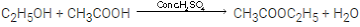75. Draw the structural formula for each of the following :
(i) 2, 3-dimethyl butane
(ii) Diethyl ether
(iii) Propanoic acid

76. Identify the term or substance based on the descriptions given below:
(i) Ice like crystals formed on cooling an organic acid sufficiently.
(ii) Hydrocarbon containing a triple bond used for welding purposes
(iii) The property by virtue of which the compound has the same molecular formula but different structural formulae.
(iv) The compound formed where two alkyl are linked by